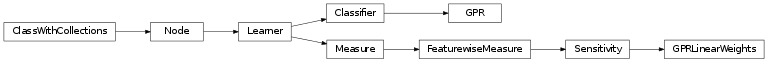# mvpa2.clfs.gpr¶

Gaussian Process Regression (GPR).Functions

 `NLAcholesky`(a) Cholesky decomposition. `NLAsolve`(a, b) Solve a linear matrix equation, or system of linear scalar equations. `Ndiag`(v[, k]) Extract a diagonal or construct a diagonal array. `Ndot`(a, b[, out]) Dot product of two arrays. `SLcho_solve`(c_and_lower, b[, overwrite_b, ...]) Solve the linear equations A x = b, given the Cholesky factorization of A. `SLcholesky`(a[, lower, overwrite_a, check_finite]) Compute the Cholesky decomposition of a matrix. `accepts_dataset_as_samples`(fx) Decorator to extract samples from Datasets. `array`(object[, dtype, copy, order, subok, ndmin]) Create an array. `asarray`(a[, dtype, order]) Convert the input to an array.

Classes

 `Classifier`([space]) Abstract classifier class to be inherited by all classifiers `ConditionalAttribute`([enabled]) Simple container intended to conditionally store the value `Dataset`(samples[, sa, fa, a]) Generic storage class for datasets with multiple attributes. `EnsureFloat`() Ensure that an input (or several inputs) are of a data type ‘float’. `EnsureNone` Ensure an input is of value `None` `EnsureRange`([min, max]) Ensure an input is within a particular range `GPR`([kernel]) Gaussian Process Regression (GPR). `GPRLinearWeights`(clf[, force_train]) `SensitivityAnalyzer` that reports the weights GPR trained `GeneralizedLinearKernel`(\*args, \*\*kwargs) The linear kernel class. `LinearKernel`(\*args, \*\*kwargs) Simple linear kernel: K(a,b) = a*b.T `Parameter`(default[, constraints, ro, index, ...]) This class shall serve as a representation of a parameter. `Sensitivity`(clf[, force_train]) Sensitivities of features for a given Classifier. `SquaredExponentialKernel`([length_scale, sigma_f]) The Squared Exponential kernel class.

Exceptions

 `Classifier`([space]) Abstract classifier class to be inherited by all classifiers `ConditionalAttribute`([enabled]) Simple container intended to conditionally store the value `Dataset`(samples[, sa, fa, a]) Generic storage class for datasets with multiple attributes. `EnsureFloat`() Ensure that an input (or several inputs) are of a data type ‘float’. `EnsureNone` Ensure an input is of value `None` `EnsureRange`([min, max]) Ensure an input is within a particular range `GPR`([kernel]) Gaussian Process Regression (GPR). `GPRLinearWeights`(clf[, force_train]) `SensitivityAnalyzer` that reports the weights GPR trained `GeneralizedLinearKernel`(\*args, \*\*kwargs) The linear kernel class. `LinearKernel`(\*args, \*\*kwargs) Simple linear kernel: K(a,b) = a*b.T `Parameter`(default[, constraints, ro, index, ...]) This class shall serve as a representation of a parameter. `Sensitivity`(clf[, force_train]) Sensitivities of features for a given Classifier. `SquaredExponentialKernel`([length_scale, sigma_f]) The Squared Exponential kernel class.